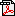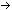# Statistics and plotting on the TI-86

## by Sam Jordan

### edited by Ray Kremer

Even I must admit that the TI-86 manualis hard to follow when it comes to using the built in statistics functions. I have reproduced here (with only minor changes for clarity) five posts made to TI's Statistics and Probability discussion group by Sam Jordan which do a much better job of explaining things. If you are doing a lot of statistics with your TI-86, you might also be interested in TI's Advanced Statistics Application which replicates the TI-83's statistical abilities. If you are using a TI-85, make sure you check the manual for the differences between it and the TI-86 described here.

Karl Parr has written another guidefor TI-86 statistics.

Back to the FAQ

#### Statistical Analysis

The first step in performing a statistical analysis on the TI-86 is determining the type of data you will be working with. The two types are single variable data sets, and paired variable data sets. On the TI-86 these are called OneVar and TwoVar (short for One variable and Two variable) data sets.

An example of a single variable data set would be the height in inches of all male students in the Statistics 101 class. Let us assume that there are 9 male students in the statistics class with the following heights measured to the nearest inch.

64, 66, 68, 69, 70, 70, 71, 72, 74

The TI-86 will perform a single variable analysis of this data if we place it in the proper "LIST" format, and store it in a list variable. By default, the TI-86 uses the list variable "xStat" for its analysis. We may save it in "xStat" via:

{64,66,68,69,70,70,71,72,74}xStat

where "" is the TI-86 "store" operator. You can also use the on-screen list editor by entering [2nd][STAT] then [F2].

Along with the "xStat" variable, we also must define a "fStat" list which gives the "frequency" of each of the values in the "xStat" list. (The TI-85 uses yStat in the place of fStat for OneVar analysis) In this case, the frequencies are all "1", and the "fStat" list is:

{1,1,1,1,1,1,1,1,1}fStat

Since at least one of the values in the "xStat" list is repeated, we could have entered the "xStat" and "fStat" lists as:

{64,66,68,69,70,71,72,74}xStat
{1,1,1,1,2,1,1,1}fStat

which in this case means that the value "70" occurs 2 times in the original list.

Assuming that we have entered these two lists, and that we have exactly the same number of entries (8) in both lists, we can now find the "OneVar" or "one variable" statistics for this set of data. All we need to do is enter the command:

OneVar

This may be done by typing it directly to the home screen, or by selecting it from the "STAT" menus by entering:
[2nd][STAT]
[F1]
[F1]
while on the home screen.

This will display the following:

1-Var Stats
x-bar=69.3333333
Ex=624
Ex2=43338
Sx=3.04138127
ox=2.86744176
n=9
minX=64
Qrtl1=67
Med=70
Qrtl3=71.5
maxX=74

Actually, I couldn't type the exact variable names that appeared in the above output, but the actual ones are described in the manual and are the:

Mean
Sum of X
Sum of squared X
Sample Standard Deviation of X
Population Standard Deviation of X
Number of data values
Minimum X value
1st Quartile
Median X
3rd Quartile
Maximum X

That pretty much covers single variable statistics.

Two variable statistics are handled in much the same way as One variable stats.

Assume we have the following (x,y) pairs:

(1,3) (2,4.1) (3,5) (4,5.9)

These would be entered into the stat lists:

{1,2,3,4}xStat
{3,4.1,5,5.9}yStat
{1,1,1,1}fStat

Notice that all three lists contain the same number of items. THIS IS IMPORTANT! If they don't contain the same number of items, you will get weird errors when trying to perform analysis of the data later!

You can obtain the two variable stats with the command "TwoVar" by either entering it on the command line, or selecting it from the [STAT] menus. TwoVar will give the following:

2-Var Stats
x-bar=2.5
Ex=10
Ex2=30
Sx=1.29099445
ox=1.11803399
n=4
y-bar=4.5
Ey=18
Ey2=85.62
Sy=1.24096736
oy=1.07470926
Exy=49.8
minX=1
maxX=4
minY=3
maxY=5.9

Again, I couldn't type the exact output, but the actual stuff is described in the manual. The values are actually stored to variables, you can use them afterward by taking the variable names from the STAT-VARS menu or the CATLG-VARS-STAT menu.

To do regressions on the same data as entered in xStat, yStat and fStat, you can use the regressions built into the [STAT] menus. For example, you can perform a linear regression on that data by "LinR".

This will produce:
LinReg
y=a+bx
a=2.1
b=.96
corr=.998700454
n=4

which indicates the line:
y=2.1+.96*x
which has a linear correlation of .998700454 with the data.

#### Statistics Command Defaults

I can't say that I've tried this, but in Chapter 20 of my TI-86 manual, it lists some shorthand ways of invoking the statistical analysis commands.

In general, if you specify nothing, the default parameters will be xStat, yStat, fStat and the equation goes into RegEq.

If the only thing you want to change is the location of the regression equation then just type the name of the equation variable. Say you want to use xStat, yStat, fStat and y3 as the regression equation storage for a linear regression. Do:
LinR y3
What I think the calculator is doing is looking at the parameters after LinR for first the list names, and then the Regression Equation name. If it doesn't see any predefined lists, then it uses all the default lists and assumes that the next parameter must be the Regression Equation name. The optional parameters vary depending on the type of regression.

There is another shortcut, this one involving fStat. If you specify the x list and y list (usually xStat and yStat) at the command line, the calculator will automatically create an fStat of the proper size, filled with all 1's.

Also, you don't have to retype those long mixed-case variable names each time you want them. Instead, you can hit [2nd][LIST] then [F3] for NAMES. Use the F-keys to paste a name to the screen and the MORE key to get the next five names. Alternatively, you can hit [2nd][CUSTOM] to get the "CATLG-VARS" menu followed by [F5] to get the list names which will include your xStat, yStat and fStat list names. Just cursor down to the item you wanted to type and hit [ENTER] to paste its name to the screen where you are working.

#### Stat Plotting

Assuming that you have data in a list:

{1,1,2,2,3,4}xStat

First select the Plot mode.
1. [2nd][STAT] and then [F3] for the plot menu to turn on a plot and select a mode. Turn on PLOT 1 by selecting PLOT1 with [F1] and use the cursor to move to blinking ON and press [ENTER]. Then cursor down to the line "Type=" and use the function keys to select the plot type that you want. For single variable data, the only valid types are MBOX or HIST or BOX. You can then cursor down again to the "Xlist Name=" line and enter the name of the list in which you have stored your data and then press enter to have it remembered. Finally you can cursor down one more time to the "Freq=" line and use either "1" to indicate that each value in your list is to be counted 1 time, or enter the name of a frequency list which MUST have the same number of entries as your single variable list. When this data is correct, you need to exit the stat menus back to the home screen.

2. You can plot your data by hitting [GRAPH][F5] which will draw the selected plot.

To plot two variable data, then instructions are similar to the above, except that the valid plot types then become either SCAT or xyLINE.

#### Regression Equation Graphs

After you've read everything above on how to enter data and calculate basic stats on it and on how to Plot the data you entered you might also want to be able to graph any Regression equation that you got during the analysis just to see how it looks against the data that you entered and plotted.

The good news is that this is fairly simple. After you've entered all your data and run your Regression function (usually "LinR" for a Linear Regression) the calculator will store the actual Regression expression into one of the Statistics variables called "RegEq". To graph it, all you need to do is go into the GRAPH menu and select "y(x)=" to enter a new graphing equation. Assuming that you use "y1=" the actual entry you make needs to be:

y1=RegEq

Now simply make sure that this selected and graph it like you would any other equation. If you want to see what the actual expression is, you can execute
[2nd][RCL] ReqEq
from the home screen.

It is often instructive to use the previously posted "Plot" instructions to generate a "Scatter Plot" of your x and y lists (for two variable statistics) and then generate and graph the Regression Equation so that they are both displayed at the same time to get a visual view of how well the equation fits the data. If you decide to try a different regression equation, all you have to do is go back to the stats menu and select the new regression function. The regression will run and store the equation into the same ReqEq variable as before, and you can then graph it.

If you DON'T want the "y1=" graph to change each time you run a new regression, then when you originally set it up, instead of entering
y1=RegEq
then at the "y1=" prompt you need to enter
[2nd][RCL] RegEq [ENTER]
to set it equal to the actual expression which won't change then next time you assign something new to RegEq by running a new regression.

#### Computing and Plotting Residuals

Residuals are defined as the difference between the "observed" and "predicted" values of a regression.

You begin with a set of x,y data pairs:
(1,3) (2,4.1) (3,5) (4,5.9)

You enter these into the x and y stat lists as:
{1,2,3,4}xStat
{3,4.1,5,5.9}yStat
{1,1,1,1}fStat

(Note that all lists have the same number of elements, and "fStat" is set to all ones. If you don't do both of these, it won't work! Also note that if you use the LIST editor to enter these, you'll need to get out of it and back into the STAT menus before you can do anything else even if you went to the LIST menu directly from the STAT menu!)

After you've entered these lists, you can use the STAT PLOT menu to plot them as a scatter plot.

You can also run "LinR" to generate their regression equation which provides the line of "best fit" to the points. After doing this, you can assign the resulting "RegEq" to one of the graph function variables so that you can graph it against your original scatter plot. The easiest way is simply to enter
y1=RegEq
on the GRAPH y(x)= menu. You can also set it equal to the actual regression equation by typing just
y1=
on the home screen, and then doing
[2nd][RCL] RegEq
to recall the actual equation value as:
y1=2.1+.96x
and then hitting enter to store it.

If you need to compute the "residual" difference between the regression equation and the original yStat values, that is done by:
yStat-y1(xStat)
which can then be stored to a list as:
yStat-y1(xStat)rStat

which in this case should be set to:
{-.06,.08,.02,-.04}

If you then want to plot the residuals, you may do so by defining a scatter plot with:
Xlist name=xStat
Ylist name=rStat

and make sure that you set your yMin and yMax values small enough based on the largest and smallest values in "rStat" so that you can see the plot.

This same technique can be used to plot the residuals of ANY regression.

Back to the FAQvisitors since 8/1/2001